# How to Convert Radians to Degrees in Excel (Easy Formula)

When working with mathematical data, there might be instances where you need to work on angles. Excel has a number of built-in trigonometric functions that let you easily calculate the cosine, sine, or tangent of angles.

The problem is that these functions give results in radians, rather than degrees.

That makes sense from mathematical and scientific points of view, but degrees are a more commonplace representation of angles. As such, it is often useful to convert angle measures from radians to degrees.

With Excel, this is quite easy and in this tutorial, we will show you three ways in which you can convert from radians to degrees. These include:

• Using a function specifically built to convert radians to degrees
• Using a formula that uses the PI() function.
• Using VBScript to quickly convert a range of cell values from radians to degrees.

For each of these methods, we will also show you how to convert degree values back to radians as well.

## Method 1: Using a Formula to Convert Radians to Degrees

Let’s say you have the following columns containing angles in radians, and you want to convert them all to degrees: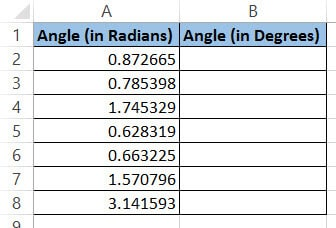The first method uses the formula that one commonly uses to convert radians to degrees. You most probably already know the formula, but even then, here’s a recap.

If rad is the value in radians and deg is the value in degrees, then to convert radians to degrees, the formula used is: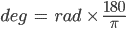If this is converted to an Excel formula, it becomes:

`= A1 * 180/PI()`

for a particular cell A1 containing an angle value in radians.

Notice that we used the PI() function in this formula. PI() is a very helpful Excel function that returns the value of the constant (pi), accurate to 15 digits.

Let us see a step-by-step on how we can apply this formula to your Excel dataset:

1. Select the first cell of the column where you want your converted values to appear. In our example, it will be cell B2.
2. Type the ‘equal to’ sign (=).
3. Next, select the first cell containing the value you want to convert (A2).
4. A reference to cell A2 should appear in cell B2. Following that, type: ‘* 180/PI()’ and press the return key.
5. This will display the value in A2 converted to its equivalent in degrees.
6. Double-click the fill handle (located at the bottom right corner of the cell B2). This will copy the formula to the rest of the cells in column B. You can also choose to drag down the fill handle to achieve the same effect.

Here are the angle values, both in radians and degrees side by side: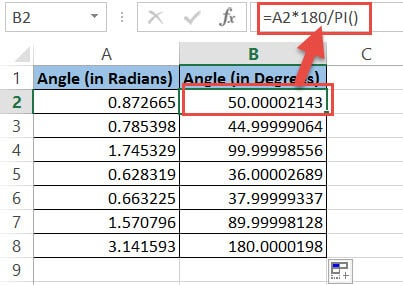Now, this method will usually give you a very accurate measure of the radian value when converted to degrees. So, if you convert an angle of 0.872664 radians, you will get a value of 49.9999641330045 in degrees.

This is very close to 50 degrees, so it would make sense to round it up to 50 rather than have so many decimals (unless you are really particular about accuracy).

For this, you can round up the result to its nearest whole number by using the ROUND function. So your formula should now be:

`=ROUND(A2 * 180/PI(),0).`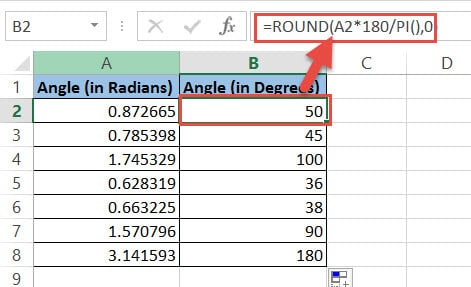If you don’t want to round up the value, you can skip this part.

So you now have a whole column of values in degrees. These values change when radian values in their corresponding cells are changed. If you want to keep both of them side by side, then you’re good.

But if you only want to keep the values in degrees, then you need to select these cells, copy them and paste them in the same place as values.

Note: If you want to convert angle values back to radians, you need to use the formula: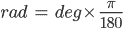So if a cell B2 contains the value in degrees that you want to convert to radians, you need to use the following Excel formula:

`= B2 * PI()/180`

## Method 2: Using a Function to Convert Radians to Degrees

Excel provides some trigonometric functions that you can directly use. There’s one to convert radian values to degrees and one to convert degree values to radians.

To convert radians to degrees, you can use the DEGREES function. The syntax for this function is:

`=DEGREES(angle)`

Here, the argument angle is where you can place either the value of an angle in radians or a cell reference containing a value in radians.

For example, to convert the value 0.872664 radians to degrees, you can type:

`=DEGREES(0.872664)`

If the value is stored in cell A1, you could type:

`=DEGREES(A1)`

In both cases, you will get the same results when you press the return key.

Let us see a step-by-step on how we can apply this function to the same Excel dataset that we used for the previous method:

1. Select the first cell of the column where you want your converted values to appear. In our example, it will be cell B2.
2. Type the ‘equal to’ sign, followed by the word ‘DEGREES’ and opening parentheses: ‘=DEGREES(‘ .
3. Next, select the first cell containing the value you want to convert (A2).
4. Close the parentheses ‘)’
5. Press the return key.
6. This will display the value in A2 converted to its equivalent in degrees.
7. Double-click the fill handle (located at the bottom right corner of the cell B2). This will copy the formula to the rest of the cells in column B. You can also choose to drag down the fill handle to achieve the same effect.

Here are the angle values, both in radians and degrees side by side: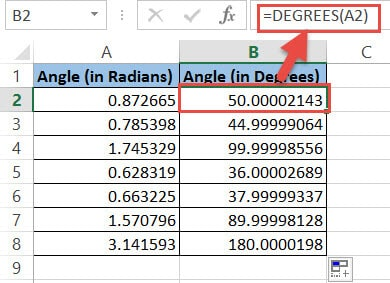Again, this method will give you a very accurate measure of the radian value when converted to degrees. So if you want to round up the result to its nearest whole number, you can use the ROUND function.

So your formula should now be: =ROUND(DEGREES(A2),0). If you don’t want to round up the value, you can skip this step.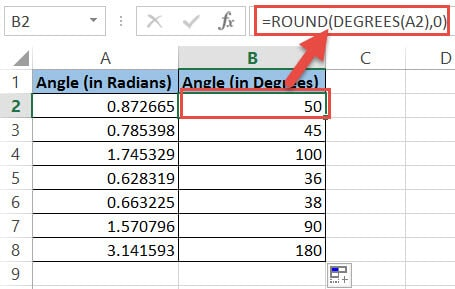You now get a whole column of values in degrees. These values change when radian values in their corresponding cells are changed.

If you want to keep only the values in degrees, then you need to select these cells, copy them and paste them in the same place as values.

Note: If you want to convert these values back to radians, you need to use Excel’s RADIANS function. The syntax for this function is:

`=RADIANS(angle).`

So if a cell B2 contains the value in degrees that you want to convert to radians, you need to type:

`= RADIANS(B2)`

## Method 3: Using VBScript to Convert Radians to Degrees

Finally, if the idea of a little coding does not intimidate you, here’s a third method that you can use.

We are going to use VBScript to convert values from radians to degrees.

If you want to replace the radian values with their corresponding degree values, you can use the following VBA code:

```Sub radians_to_degrees()
For Each cell In Selection
cell.Value = Application.WorksheetFunction.Degrees(cell.Value)
Next cell
End Sub```

This code takes a selection of cells and one by one converts them to degrees, replacing the original value with the converted value.

Note that this method permanently changes the values in your worksheet. So there’s no way that you can undo it once the change is made.

If, however, you want to retain the original values, you can have the converted values displayed in the next column instead. For this, use the following code:

```Sub radians_to_degrees()
For Each cell In Selection
cell.Offset(0, 1).Value = Application.WorksheetFunction.Degrees(cell.Value)
Next cell
End Sub```

1. From the Developer Menu Ribbon, select Visual Basic.
2. Once your VBA window opens, Click Insert->Module. Copy the code given above and paste it into the module window.
3. Your code is now ready to run and use whenever needed. Close the VBA window.
4. Now go to your worksheet and select the group of cells containing angle values in radians.
5. Click on Macros, under the Developer tab.
6. This will open the Macros dialog box. Select the name radians_to_degrees from the list of macros displayed.
7. Click OK.

Your code should now run and you should see the results on your worksheet.

If you need to make conversions like this all the time, you can create a quick access button to quickly run the conversion macro whenever you need it.

Note: To convert from degrees to radians, you can use this same code, by just changing the function name ‘degrees’ in line 3 to ‘radians’. So your line 3 should be:

`cell.Offset(0, 1).Value = Application.WorksheetFunction.Radians(cell.Value)`

These were three simple and quick ways to convert angle values from radians to degrees and or degrees to radians.

So you see, you don’t need scientific calculators or third party apps for this simple process because Excel provides enough ways for you to get your conversions done easily.

Other Excel tutorials you may find useful: